# Catch up on the latest AI articles# Fast NAS Method Using Batch Regularization

3 main points
✔️ Proposed a batch regularization-based metric for architecture evaluation to reduce the evaluation cost
✔️ Reduced learning cost by learning only batch regularization layer even for supernet
✔️ Achieve higher speedup in training and search phases without loss of accuracy

written by Boyu ChenPeixia LiBaopu LiChen LinChuming LiMing SunJunjie YanWanli Ouyang
(Submitted on 16 Aug 2021)

Subjects: Computer Vision and Pattern Recognition (cs.CV)code：The images used in this article are from the paper, the introductory slides, or were created based on them.

## first of all

Neural Architecture Search (NAS) is a general term for methods to search neural network architectures automatically. Recently, various types of research have been done and it has become possible to search architectures with high accuracy, but there are still many issues to be solved. One of the major issues is the cost of the search. The cost of evaluating candidate architectures in search is very high, so research is being done on reducing the cost. In this paper, we try to reduce the cost by using batch regularization parameters as a network evaluation metric and supernet learning.

## background knowledge

### one-shot NAS

It is a method to search a large supernet for a subnet that fits the desired task. The entire pipeline of the method can be divided into the following three stages

1. Learning Supernet
2. Subnet Search
3. Subnet relearning

#### Learning Supernet

Supernets have multiple Operators at each layer as shown in the figure below.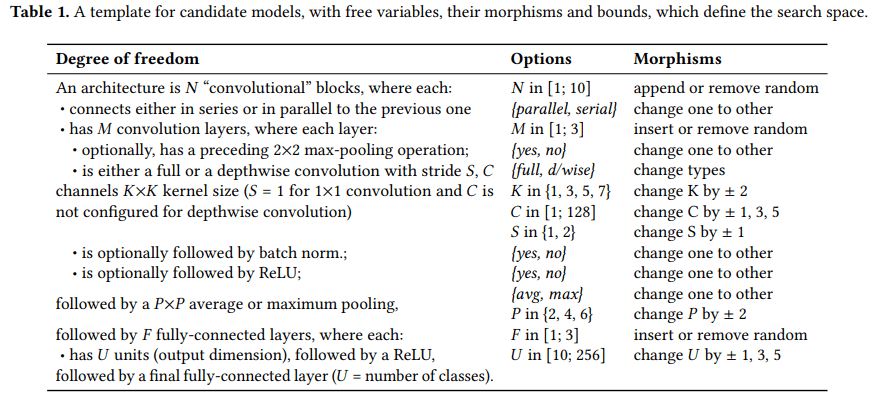Since we cannot learn the weights of all these candidate operations in a single error backpropagation, we sample the single-pass architecture (Op3 → Op2 → Op3 in the figure) based on the sampling policy at each iteration before training. Since we sample this at each iteration, the entire network is learned over the iterations.

#### subnet searching

Once the supernets are trained, the next step is to search for the best performing optimal architecture among them. We often use the accuracy in the validation data to evaluate the subnets.

#### Subnet relearning

In the re-training phase, the K most accurate subnets are re-trained in the subnet search phase. Then they are evaluated on the validation data and the most precise subnet is selected as the final optimal subnet.

### batch regularization layer

The batch regularization layer is used for network pruning and is considered suitable for evaluating the importance of channels. Let Xin be the input to the batch regularization layer, the output Xout of the batch regularization layer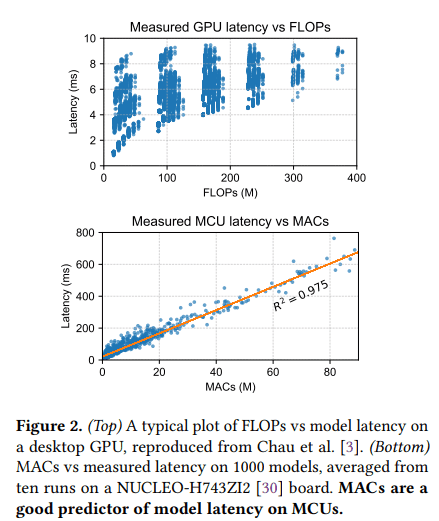is calculated as follows. The parameters in the equation that are updated when the network is trained are β and γ.

## proposed method

### outline

The framework of the proposed method is outlined in the following figure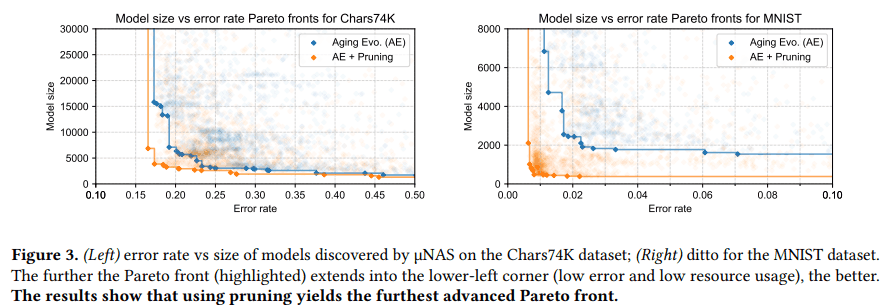The method is based on the one-shot NAS. There are two differences

1. In the learning phase of the supernet, the convolution parameters are fixed and only the parameters of the batch regularization layer are learned.
2. In the subnet search phase, the proposed batch regularization-based metrics are used to find subnets

We now describe the batch regularization-based metrics used in the subnet search phase.

As a restatement, the following figure describes the batch regularization-based indicators.In one-shot NAS, as mentioned earlier, we define an Operation (Op in the figure) in each layer, and the breakdown of the Operation is shown in the middle of the figure. In the proposed method, we only use the parameters of the last batch regularization layer in this Operation. Therefore, it is not possible to select an Operation that does not have a batch regularization layer at the end, but most of the existing NAS methods have a batch regularization layer at the end, so the proposed method can be applied.

The indicator using batch regularization consists of two parts. It is

1. Evaluation of Operation by Batch Regularization Layer
2. Evaluation of the architecture by 1.

The following is a list of the most important features of the system. These are explained in the following sections.

### Evaluation of Operation by Batch Regularization

The evaluation value of the Operation of each layer is calculated as the following formula.In this equation, Operation is evaluated using γ, which is a learnable parameter in batch regularization.

### Evaluate the architecture using each Operation evaluation

Each Operation evaluation is obtained as in the formula above, and then they are combined to calculate the architecture's evaluation value. Specifically, it is calculated according to the following formula.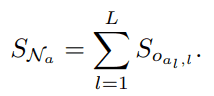## experiment

### Comparison with the baseline method

As a baseline, we choose the SPOS and FairNAS methods. The comparison results are shown in the table below.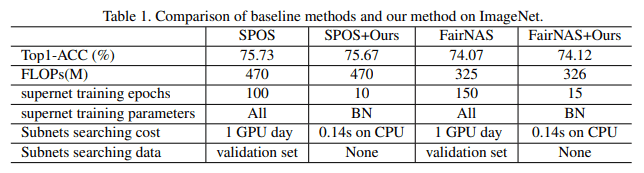It can be seen that the proposed method can significantly reduce the computational cost in SPOS and FairNAS without any loss of accuracy.

### Comparison with SOTA methods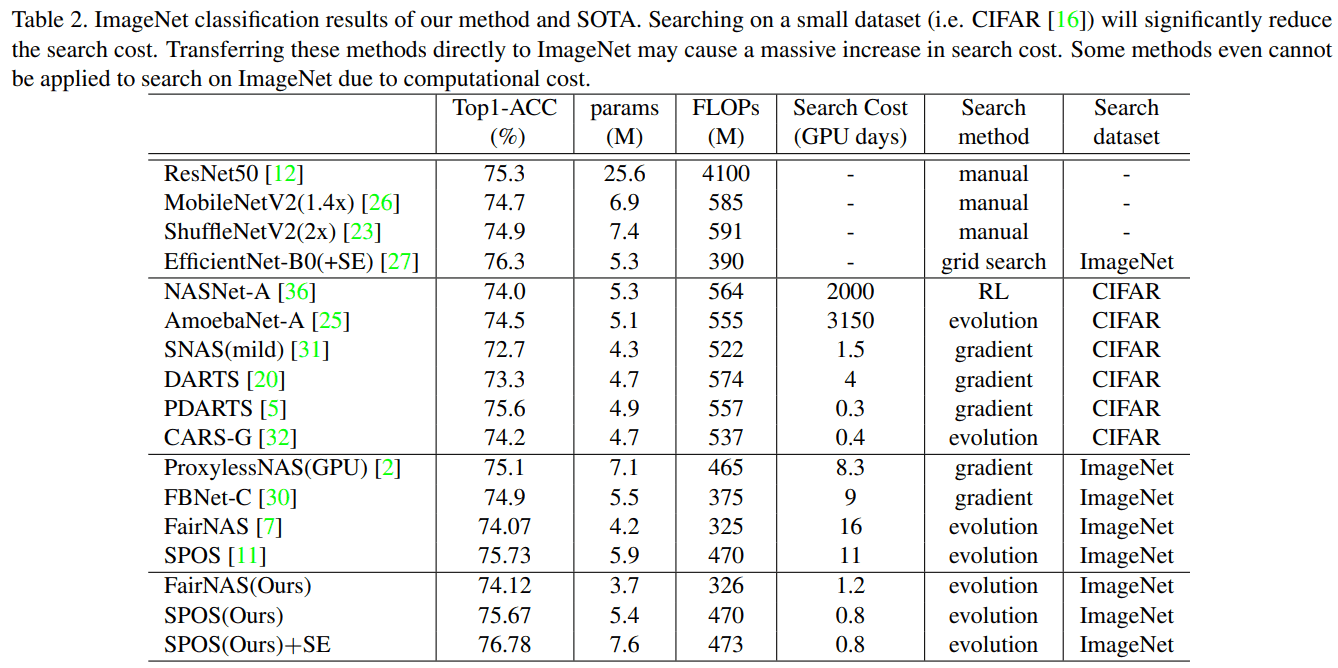The comparison results with the SOTA method are shown in the above table. It can be seen that the proposed method combined with SPOS achieves higher performance with less number of FLOPs when compared with the manually designed network. It can also be seen that the proposed method achieves higher performance with less number of FLOPs as compared to the NAS method in SOTA. As for the search cost, it can be seen that the proposed method requires less than 1/10th of the search cost compared to the method that directly searches the architecture.

## summary

To solve the problem of search cost, which is one of the major issues in NAS, the proposed method tries to reduce the search cost by using only the batch regularization layer as a learning and evaluation metric. Since the supernet is learned only by the batch regularization layer, the learning time of the supernet is shortened and the subnet can be efficiently explored by evaluating the subnet using the parameters of the batch regularization layer.

If you have any suggestions for improvement of the content of the article,
please contact the AI-SCHOLAR editorial team through the contact form.